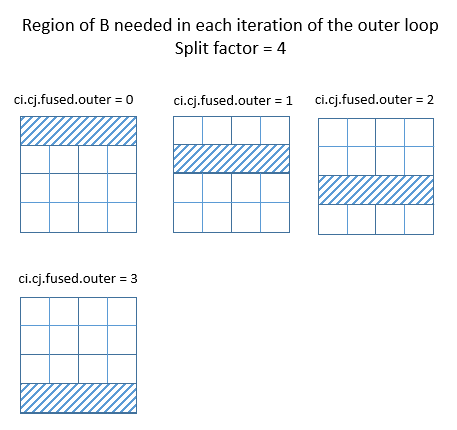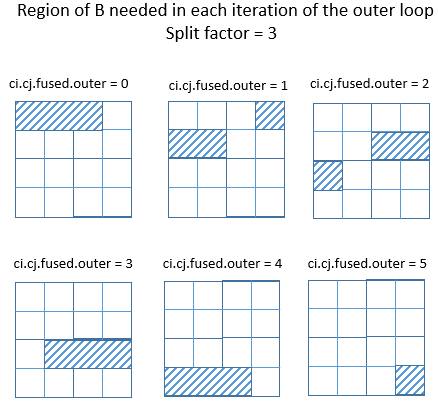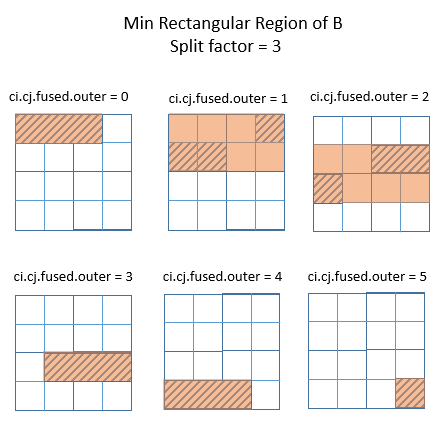# InferBound Pass

InferBound pass 在 normalize 之后、ScheduleOps build_module.py 之前运行。InferBound 的主要工作是创建 bounds map，为程序中的每个 IterVar 指定一个 Range。接下来这些 bounds 会传递给 ScheduleOps，用于设置 For 循环的范围，参阅 MakeLoopNest，以及设置分配缓冲区的大小（BuildRealize）以及其他用途。

InferBound 的输出是从 IterVar 到 Range 的映射：

``Map<IterVar, Range> InferBound(const Schedule& sch);``

``namespace HalideIR {namespace IR {     class RangeNode : public Node {     public:             Expr min;             Expr extent;             // 剩余部分省略     };     }}namespace tvm {     class IterVarNode : public Node {     public:             Range dom;             Var var;             // 剩余部分省略     };}``

InferBound 接收一个参数，即 Schedule。这个 schedule 对象及其成员包含正在编译的程序的所有信息。

TVM schedule 由 stage 组成。每个 stage 只有一个 Operation，例如 ComputeOp 或 TensorComputeOp。每个 Operation 都有一个 root_iter_vars 列表，在 ComputeOp 的情况下，它由 axis IterVar 和 reduce axis IterVar 组成。

``namespace tvm {     class ScheduleNode : public Node {     public:             Array<Operation> outputs;             Array<Stage> stages;             Map<Operation, Stage> stage_map;             // 剩余部分省略     };     class StageNode : public Node {     public:             Operation op;             Operation origin_op;             Array<IterVar> all_iter_vars;             Array<IterVar> leaf_iter_vars;             Array<IterVarRelation> relations;             // 剩余部分省略     };     class OperationNode : public Node {     public:             virtual Array<IterVar> root_iter_vars();             virtual Array<Tensor> InputTensors();             // 剩余部分省略     };     class ComputeOpNode : public OperationNode {     public:             Array<IterVar> axis;             Array<IterVar> reduce_axis;             Array<Expr> body;             Array<IterVar> root_iter_vars();             // 剩余部分省略     };}``

``class TensorNode : public Node {public:     // 源操作，可以是 None     // 这个 Tensor 是这个 op 输出的     Operation op;     // 源操作的输出索引     int value_index;};``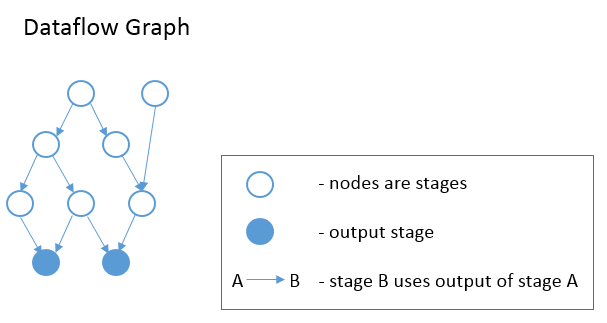InferBound 使 pass 遍历计算图，每个 stage 访问一次。InferBound 从输出 stage 开始（即上图中的实心蓝色节点），然后向上移动（在边缘的相反方向上）。这是通过对计算图的节点执行反向拓扑排序来实现的。因此，当 InferBound 访问一个 stage 时，它的每个 consumer stage 都已经被访问过。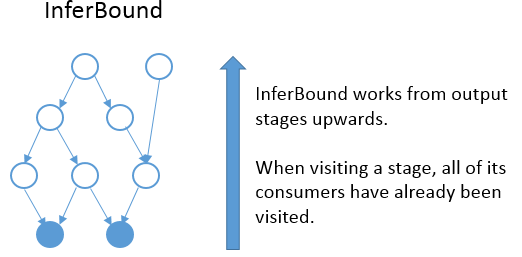InferBound pass 如以下伪代码所示：

``Map<IterVar, Range> InferBound(const Schedule& sch) {     Array<Operation> outputs = sch->get_outputs();     G = CreateGraph(outputs);     stage_list = sch->reverse_topological_sort(G);     Map<IterVar, Range> rmap;     for (Stage s in stage_list) {             InferRootBound(s, &rmap);             PassDownDomain(s, &rmap);     }     return rmap;}``

InferBound pass 有两个不是很明显的属性：

1. InferBound 访问一个 stage 后，stage 中所有 IterVar 的范围都会在 `rmap` 中设置。
2. 每个 IterVar 的 Range 只在 `rmap` 中设置一次后就不会再变了。

## IterVar Hyper-graph​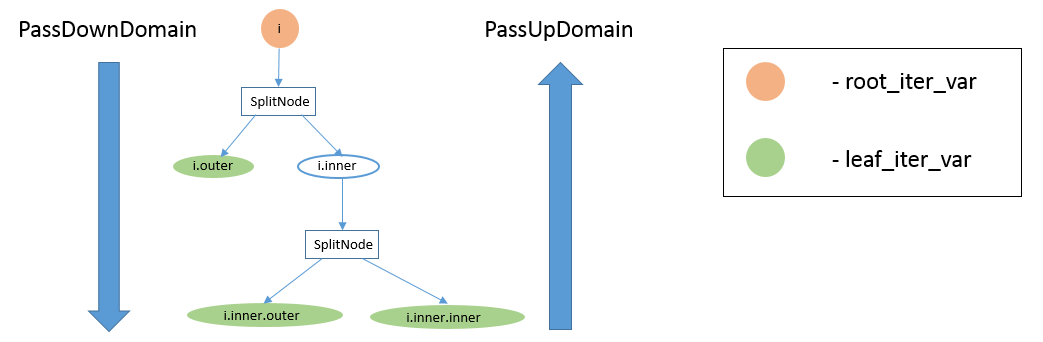## PassDownDomain​

PassDownDomain 的作用是为 root_iter_vars 取 InferRootBound 产生的 Range，并设置 stage 中所有其他 IterVars 的 Range。

PassDownDomain 遍历 stage 的 IterVarRelations。IterVarRelation 有三种可能的类型：split、fuse 和 rebase。最有趣的案例（因为它还有改进空间）是表示 split 的 IterVarRelations。

``rmap[split->inner] = Range::FromMinExtent(0, split->factor)rmap[split->outer] = Range::FromMinExtent(0, DivCeil(rmap[split->parent]->extent, split->factor))``

`split->factor` 没有平均划分父节点的范围时，就有机会收紧 InferBound 产生的边界。假设 parent 的范围是 20，split 因子是 16。那么在外部循环的第二次迭代中，内部循环只需要进行 4 次迭代，而非 16 次。如果 PassDownDomain 可以设置 `split->inner` 的范围为 `min (split->factor, rmap[split->parent]->extent - (split->outer * split->factor))`，则内部变量的范围将根据正在执行的外部循环的迭代进行适当调整。

``rmap[fuse->fused] = Range::FromMinExtent(0, rmap[fuse->outer]->extent * rmap[fuse->inner]->extent)``

## InferRootBound​

InferBound 调用 InferRootBound，然后在 stage 计算图中的每个 stage 调用 PassDownDomain。InferRootBound 的目的是设置 Stage 操作的每个 root_iter_var 的 Range。这些 Range 会用 PassDownDomain 传到 Stage 的其余 IterVars。注意，InferRootBound 不会设置任何其他 IterVar 的 Range，仅设置属于 Stage 的 root_iter_vars 的那些。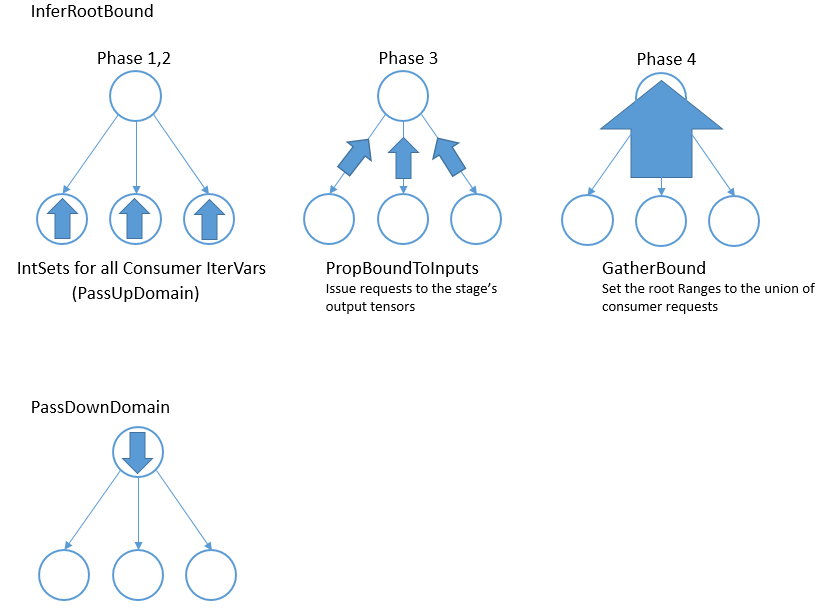### 阶段 1：为 consumer 的 leaf_iter_vars 初始化 IntSet​

``/* * 输入: Map<IterVar, Range> rmap: 包含 consumer stage 的每个 IterVar 的 Range * 输出: Map<IterVar, IntSet> up_state: 包含 consumer 的每个 leaf_iter_var 的 IntSet */``

• 案例 1：leaf var 的 Range 范围为 1。这种情况下，leaf 的 up_state 只是一个点，等于 Range 的最小值。
• 案例 2：不需要释放。这种情况下，leaf 的 up_state 只是一个点，由 leaf var 本身定义。
• 案例 3：需要释放。这种情况下，leaf 的 Range 被简单地转换为 IntSet。

### 阶段 2：将 IntSet 从 consumer 的 leaf 传到 consumer 的 root​

``/* * Input: Map<IterVar, IntSet> up_state: consumer leaf -> IntSet * Output: Map<IterVar, IntSet> dom_map: consumer root -> IntSet */``

• 案例 1：外部和内部 IterVar 的范围匹配它们的 `up_state` 域。在这种情况下，只需将父级的 Range 转换为 IntSet 即可设置父级的 `up_state`
• 案例 2：否则，父级的 `up_state` 是相对于外部和内部的**`up_state`通过评估 `outerf + inner + rmap[parent]->min`*来定义的。这里，TVM 没有使用*s**plit 关系的因子，而是用*`f = rmap[inner]->extent`。

``dom_map[iv->var.get()] = IntSet::range(up_state.at(iv).cover_range(iv->dom));``

### 阶段 3：将 IntSet 传到 consumer 的输入张量​

``/* * Input: Map<IterVar, IntSet> dom_map: consumer root -> IntSet * Output: Map<Tensor, TensorDom> tmap: output tensor -> vector<vector<IntSet> > */``

PropBoundToInputs 的具体行为取决于 consumer 操作的类型：ComputeOp、TensorComputeOp、PlaceholderOp、ExternOp 等。TensorComputeOp 的每个张量输入都有一个区域，定义了操作所依赖的张量切片。对于每个输入张量 i 和维度 j，根据 Region 中的相应维度向 tmap 添加一个请求：

``for (size_t j = 0; j < t.ndim(); ++j) {     // i selects the Tensor t     tmap[i][j].push_back(EvalSet(region[j], dom_map));}``

### 阶段 4：整合所有 consumer​

``/* * Input: Map<Tensor, TensorDom> tmap: output tensor -> vector<vector<IntSet> > * Output: Map<IterVar, Range> rmap: rmap is populated for all of the stage's root_iter_vars */``

ComputeOp 只有一个输出张量，其轴与 ComputeOp 的轴变量一一对应。ComputeOp 的 root_iter_vars 包括这些轴变量，以及 reduce_axis 变量。若 root IterVar 是一个轴变量，它对应一个输出张量的轴。 GatherBound 将此类 root IterVar 的 Range 设置为张量相应轴的所有 IntSet 的并集（即所有 consumer 请求的并集）。如果 root IterVar 是一个 reduce_axis，它的 Range 只是设置为其默认值（即 IterVarNode 的 `dom` 成员）。

``// 'output' 选择输出张量// i 是维度rmap[axis[i]] = arith::Union(tmap[output][i]).cover_range(axis[i]->dom);``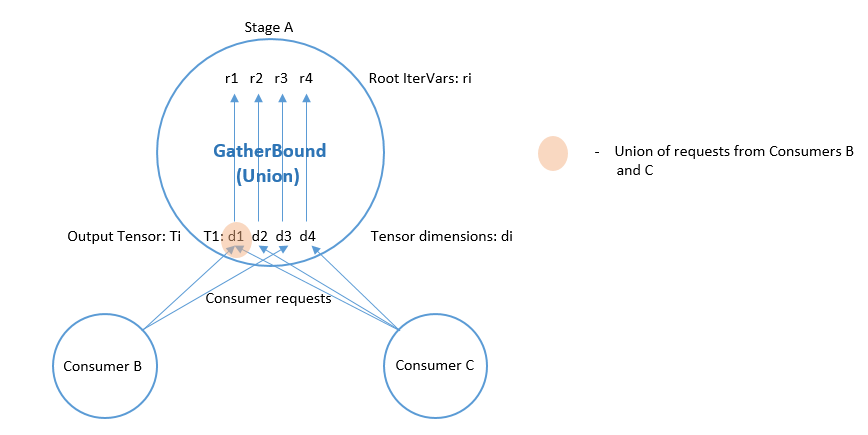IntSet 的并集是通过将每个 IntSet 转换为一个区间来计算的，然后取所有最小值中的最小值，以及所有这些区间最大值中的最大值。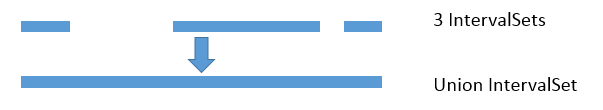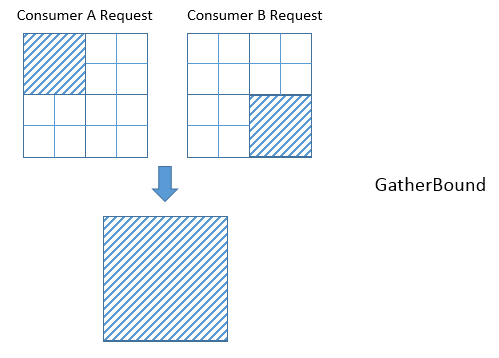## InferBound 与 compute_at​

### 动机​

``C = tvm.compute((5, 16), lambda i, j : tvm.const(5, "int32"), name='C')D = tvm.compute((5, 16), lambda i, j : C[i, j]*2, name='D')``

``for i 0, 5    for j 0, 16        C[i, j] = 5for i 0, 5    for j 0, 16        D[i, j] = C[i, j]*2``

``s = tvm.create_schedule(D.op)s[C].compute_at(s[D], D.op.axis)``

``for i 0, 5    for j 0, 16        C = 5        D[i, j] = C*2``

``for i 0, 5    for j 0, 16        C[j] = 5    for j 0, 16        D[i, j] = C[j]*2``

### 附加路径​

``class StageNode : public Node {public:    // 省略    // 对于compute_at，attach_type = kScope    AttachType attach_type;    // 对于 compute_at，这是轴    // 传递给 compute_at，例如 D.op.axis    IterVar attach_ivar;    // 传递给 compute_at 的阶段，例如 D    Stage attach_stage;    // 省略};``

CreateAttachPath 负责确定哪些作用域包含 stage C。这些作用域按从最内层到最外层的顺序排列。因此，对于每个 stage，CreateAttachPath 都会生成一个「附加路径」，其中列出了包含该 stage 从最里面到最外面的范围，在例 1，C 的附加路径为空。在例 2，C 的附加路径包含 {j，i}。在例 3，C 的附加路径是 {i}。

``C = tvm.compute((5, 16), lambda i, j : tvm.const(5, "int32"), name='C')D = tvm.compute((4, 5, 16), lambda di, dj, dk : C[dj, dk]*2, name='D')s = tvm.create_schedule(D.op)s[C].compute_at(s[D], D.op.axis)``

``realize D([0, 4], [0, 5], [0, 16]) {  produce D {    for (di, 0, 4) {      for (dj, 0, 5) {        for (dk, 0, 16) {          realize C([dj, 1], [dk, 1]) {            produce C {              for (i, 0, 1) {                for (j, 0, 1) {                  C((i + dj), (j + dk)) =5                }              }            }            D(di, dj, dk) =(C(dj, dk)*2)          }        }      }    }  }}``

``C = tvm.compute((5, 16), lambda i, j : tvm.const(5, "int32"), name='C')D = tvm.compute((5, 16), lambda i, j : C[i, j]*2, name='D')s = tvm.create_schedule(D.op)d_o, d_i = s[D].split(D.op.axis, factor=8)s[C].compute_at(s[D], d_i)``

``for i 0, 5    for j_outer 0, 2        for j_inner 0, 8            C = 5            D[i, j_outer*8 + j_inner] = C*2``

### 构建附加路径​

``C = tvm.compute((5, 16), lambda ci, cj : tvm.const(5, "int32"), name='C')D = tvm.compute((5, 16), lambda di, dj : C[di, dj]*2, name='D')E = tvm.compute((5, 16), lambda ei, ej : D[ei, ej]*4, name='E')s = tvm.create_schedule(E.op)s[C].compute_at(s[D], D.op.axis)s[D].compute_at(s[E], E.op.axis)``

`debug_keep_trivial_loop=True` 时，C 的附加路径为 {dj,di,ej,ei}，D 的附加路径为 {ej,ei}：

``// attr [D] storage_scope = "global"allocate D[int32 * 1]// attr [C] storage_scope = "global"allocate C[int32 * 1]produce E {  for (ei, 0, 5) {    for (ej, 0, 16) {      produce D {        for (di, 0, 1) {          for (dj, 0, 1) {            produce C {              for (ci, 0, 1) {                for (cj, 0, 1) {                  C[(ci + cj)] = 5                }              }            }            D[(di + dj)] = (C[(di + dj)]*2)          }        }      }      E[((ei*16) + ej)] = (D*4)    }  }}``

### 阶段 1：为 consumer 的 leaf_iter_vars 初始化 IntSet​

``/* * 输入：Map<IterVar, Range> rmap: contains the Range for each IterVar of the consumer stage * 输出：Map<IterVar, IntSet> up_state: contains an IntSet for each leaf_iter_var of the consumer */``

• 案例 1：leaf var 的 Range 范围为 1。这种情况下，leaf 的 up_state 只是一个点，等于 Range 的最小值。
• 案例 2：不需要释放。这种情况下，leaf 的 up_state 只是一个点，由 leaf var 本身定义。
• 案例 3：需要释放。这种情况下，leaf 的 Range 被简单地转换为 IntSet。 若在 consumer 中遇到 stage C 的附着点，就会发生案例 2。对于此 attach_ivar，以及 consumer 的所有更高叶变量，将应用案例 2。若 C 在叶变量的 Range 内，这将确保仅请求叶变量范围内的单个点。

### 阶段 2：将 IntSet 从 consumer 的 leaf 传到 consumer 的 root​

``/* * Input: Map<IterVar, IntSet> up_state: consumer leaf -> IntSet * Output: Map<IterVar, IntSet> dom_map: consumer root -> IntSet */``

• 案例 1：外部和内部 IterVar 的 Range 匹配它们的 `up_state` 域。在这种情况下，只需将父级的 Range 转换为 IntSet 即可设置父级的 `up_state`
• 案例 2：否则，父级的 `up_state` 是通过评估 `outer*f + inner + rmap[parent]->min` 来定义的，相对于外部和内部的 `up_state`。在这里，TVM 没有使用 split 关系的因子，而是使用* `f = rmap[inner]->extent`

PassUpDomain 将 up_state 向 consumer 传给所有 IterVars 后，将创建一个从 root_iter_vars 到 IntSet 的新映射。若 stage 没有附加到当前 consumer，那么对于 consumer 的 attach_path 中的每个变量 iv，将 iv 的 Range 添加到一个 `relax_set`。stage 的 root 变量是根据这个 `relax_set` 进行评估的。

``C = tvm.compute((5, 16), lambda ci, cj : tvm.const(5, "int32"), name='C')D = tvm.compute((5, 16), lambda di, dj : C[di, dj]*2, name='D')E = tvm.compute((5, 16), lambda ei, ej : D[ei, ej]*4, name='E')s = tvm.create_schedule(E.op)s[D].compute_at(s[E], E.op.axis)``
``for ci 0, 5    for cj 0, 16        C[ci, cj] = 5for ei 0, 5    for ej 0, 16        D = C[ei, ej]*2        E[ei, ej] = D*4``

### PassUpDomain 的限制​

``import tvmfrom tvm import ten = 4m = 4A = te.placeholder((n, m), name='A')B = te.compute((n, m), lambda bi, bj: A[bi, bj]+2, name='B')C = te.compute((n, m), lambda ci, cj: B[ci, cj]*3, name='C')s = te.create_schedule(C.op)fused_axes = s[C].fuse(C.op.axis, C.op.axis)xo, xi = s[C].split(fused_axes, 4)s[B].compute_at(s[C], xo)print(tvm.lower(s, [A, C], simple_mode=True))``

``// attr [B] storage_scope = "global"allocate B[float32 * 16]produce C {  for (ci.cj.fused.outer, 0, 4) {    produce B {      for (bi, 0, 4) {        for (bj, 0, 4) {          B[((bi*4) + bj)] = (A[((bi*4) + bj)] + 2.000000f)        }      }    }    for (ci.cj.fused.inner, 0, 4) {      C[((ci.cj.fused.outer*4) + ci.cj.fused.inner)] = (B[((ci.cj.fused.outer*4) + ci.cj.fused.inner)]*3.000000f)    }  }}``

``// attr [B] storage_scope = "global"allocate B[float32 * 4]produce C {  for (ci, 0, 4) {    produce B {      for (bj, 0, 4) {        B[bj] = (A[((ci*4) + bj)] + 2.000000f)      }    }    for (cj, 0, 4) {      C[((ci*4) + cj)] = (B[cj]*3.000000f)    }  }}``

InferBound 的任务是确定必须计算的 B 的数量。但是，在这种情况下，InferBound 为 B 的 root_iter_vars 返回的范围太大：对于 `bi``bj` 都是 `[0, 4]`。这是因为 PassUpDomain 对 Fuse 关系的限制，后续将进行详细解释。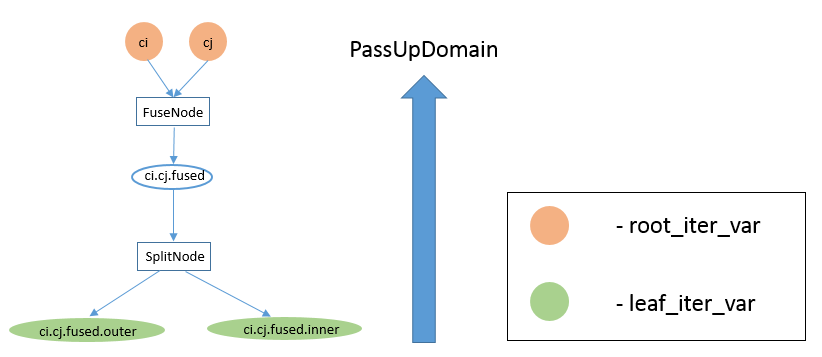IterVarIntSet**after Phase 1**
ci.cj.fused.inner[0, (min(4, (16 - (ci.cj.fused.outer*4))) - 1)]
ci.cj.fused.outer[ci.cj.fused.outer, ci.cj.fused.outer]

PassUpDomain 首先在 C 的 Split 节点上调用。PassUpDomain 的案例 2 适用，因为 `ci.cj.fused.outer` 的 IntSet 只是一个点，并且不等于它的 Range（如先前在 stage C 上由 InferBound 计算的那样）。因此，PassUpDomain 根据 `ci.cj.fused.inner``ci.cj.fused.outer` 的 IntSet 设置 `ci.cj.fused` 的 IntSet，如下表第 3 行所示。

IterVarIntSet**after PassUpDomain on SplitNode**
ci.cj.fused.inner[0, (min(4, (16 - (ci.cj.fused.outer*4))) - 1)]
ci.cj.fused.outer[ci.cj.fused.outer, ci.cj.fused.outer]
ci.cj.fused[(ci.cj.fused.outer4), ((ci.cj.fused.outer4) + (min(4, (16 - (ci.cj.fused.outer*4))) - 1))]

• 案例 1：IterVar`fused` 的 Range（如先前由 InferBound 计算的那样）等于其 IntSet
• 案例2：IterVar `fused` 的 IntSet 是单点
• 案例3：其他情况

IterVarIntSet**after PassUpDomain on FuseNode**
ci.cj.fused.inner[0, (min(4, (16 - (ci.cj.fused.outer*4))) - 1)]
ci.cj.fused.outer[ci.cj.fused.outer, ci.cj.fused.outer]
ci.cj.fused[(ci.cj.fused.outer4), ((ci.cj.fused.outer4) + (min(4, (16 - (ci.cj.fused.outer*4))) - 1))]
ci[0, 4]
cj[0, 4]